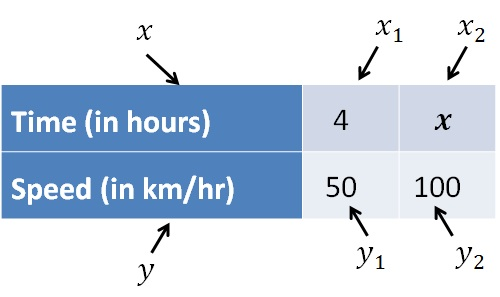Inverse Proportion

Chapter 11 Class 8 Direct and Inverse Proportions
Concept wise

Inverse proportion means

When one quantity increases, the other quantity decreases

Example

• If speed increases, time taken decreases
• If number workers doing a job increase, the total time to complete a job decreases

So, we can say that

Speed and Time are inversely proportional

Similarly,

Number of workers and total time are inversely proportional

## How to solve questions with Inverse Proportion?

Let’s take an example

### Suppose at 50 km/hr, it takes us 4 hours to reach a destination. How much time would it take to reach a destination if speed is 100 km/hr?

Given that,

It takes 4 hours to reach destination at speed of 50 km/hr

We need to find how much time would it take if speed is 100 km/hr

Let the time taken be x hours

Now, we draw a table

 Time (in hours) 4 x Speed (in km/ hr ) 50 100

Now, as speed increases

time taken will decreases

So, Time and Speed are in inverse proportion

So, we write numbers as

x 1 y 1 = x 2 y 2So,

x 1 y 1 = x 2 y 2

4 × 50 = x  × 100

(4 × 50)/100 = x

4/2 = x

2 = x

x = 2

So, it takes 2 hours to travel

Let’s take another example

### Suppose 5 workers complete a job in 10 days. How much time would it take if there are 10 workers?

Given that,

5 workers complete a job in 10 days

We need to find much time would it take if there are 10 workers

Let the time taken be y days

Now, we draw a table

 Number of workers 5 10 Time taken (days) 10 y

Now, as number of workers increases,

time taken to complete the job will decrease

So, they are in inverse proportion

So, we write numbers as

x 1 y 1 = x 2 y 2

5 × 10 = 10 × y

(5 × 10)/10=y

5 = y

y = 5

So, it will take 5 days

Learn in your speed, with individual attention - Teachoo Maths 1-on-1 Class